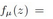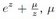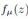##### Singular Values of a Family of Singular Perturbed Exponential Map

Journal of Advances in Mathematics and Computer Science, Page 1678-1681
DOI: 10.9734/BJMCS/2014/9598

The singular values of the one parameter family of singular perturbed exponential mapis non-zero real, are investigated. It is found that the functionhas inﬁnitely many singular values and all these singular values are bounded.

##### Centroid-Based and Bayesian Algorithms Performance

Ghaleb Al-Gaphari, Fadl M. Ba-Alwi, Saeed Abdullah M. Al Dobai

Journal of Advances in Mathematics and Computer Science, Page 1642-1664
DOI: 10.9734/BJMCS/2014/7897

Since, the amount of textual information available on the web is estimated by terra bytes. Then, there should be an efficient algorithm to summarize such information. The algorithm would speed up the process of information reading, information accessing and decision making process. This paper investigates Bayesian classifier (BC) and a Centroid -Based algorithm (CBA) performance in terms of Arabic text summarization problem (ATS). Both algorithms are implemented as a software program. The Centroid -Based algorithm (CBA) extracts the most important sentences in a document or a set of documents (cluster). This algorithm starts computing the similarity between two sentences and evaluating the centrality of each sentence in a cluster based on centrality graph. Then the algorithm extracts the most important sentences in the cluster to include them in a summary. Whereas the Bayesian algorithm categorizes each sentence to be in text summary or out of text summary classes depends on its features vector. Both algorithms are evaluated by human participants and by an automatic metrics. Arabic NEWSWIRE-a corpus is used as a data set in the algorithms evaluation. The F-measure is obtained for both algorithms results. The Centroid -Based algorithm records 0.7199 and the Bayesian algorithm records 0.623.Thereforethe Centroid -Based algorithm (CBA) outperforms the Bayesian algorithm. The CBA results show that, the CBA is a robust algorithm compared to BC. It show a low deviation average that means the CBA gives similar result either contains bugs or not compared to BC. It is able to compress or reduce the text into 25% of its original size without losing the main idea behind the original text. This property makes the algorithm distinguishable among others used for the same purpose. Also, it outperforms all those techniques which are included in this paper when it is used for Arabic text summarization.

##### Energy Decay Rate for Bresse System with Nonlinear Localized Damping

Donghao Li, Chenxia Zhang, Qingying Hu, Hongwei Zhang

Journal of Advances in Mathematics and Computer Science, Page 1665-1677
DOI: 10.9734/BJMCS/2014/9125

In this paper, we study the energy decay rate for the Bresse system in a one-dimensional bounded domain with nonlinear localized damping acting in all the three wave equations. We show the asymptotic stability without impose conditions about the equal-speed wave propagation using a method developed by Kormornik  and Martinez, providing a larger class for non-linear functions.

##### Some Lacunary Sequence Spaces of Invariant Means Defined by Musielak-Orlicz Functions

M. Aiyub

Journal of Advances in Mathematics and Computer Science, Page 1682-1692
DOI: 10.9734/BJMCS/2014/5201

The purpose of this paper is to introduce and study some sequence spaces which are defined by combining the concepts of sequences of Musielak-Orlicz functions, invariant means and lacunary convergence. We establish some inclusion relations between these spaces under some conditions. This study generalized some results .

##### Sinc-collocation Algorithm for Solving Nonlinear Fredholm Integro-differential Equations

Journal of Advances in Mathematics and Computer Science, Page 1693-1700
DOI: 10.9734/BJMCS/2014/8247

This paper is concerned with the numerical solution using sinc-collocation method of nonlinear Fredholm integro-differential equations. The algorithm is based on replacing the exact solution by a linear combination of sinc functions. The resulting nonlinear equations are treated using Newton method. Numerical examples illustrate the pertinent features of the method and its applicability to a large variety. The examples include convolution type, singular as well as singularly-perturbed problems.

##### Co-∗n-tuple of Contravariant Functors

S. Al-Nofayee

Journal of Advances in Mathematics and Computer Science, Page 1701-1709
DOI: 10.9734/BJMCS/2014/9700

In this work we generalize the concept of Co-∗n-modules to the concept of Co-n-tuple of Contravariant Functors between abelian categories.

##### Statistical Inference for Kumaraswamy Distribution Based on Generalized Order Statistics with Applications

M. M. Sharaf El-Deen, G. R. AL-Dayian, A. A. EL-Helbawy

Journal of Advances in Mathematics and Computer Science, Page 1710-1743
DOI: 10.9734/BJMCS/2014/9193

In this paper, non-Bayesian and Bayesian approaches are used to obtain point and interval estimation of the shape parameters, the reliability and the hazard rate functions of the Kumaraswamy distribution. The estimators are obtained based on generalized order statistics. The symmetric and asymmetric loss functions are considered for Bayesian estimation. Also, maximum likelihood and Bayesian prediction for a new observation are found. The results have been specialized to Type II censored data and the upper record values. Comparisons are made between Bayesian and non-Bayesian estimates via Monte Carlo simulation. Moreover, the results are applied on real hydrological data.

##### Augmented Lagrangian Method for One Dimensional Optimal Control Problems Governed by Delay Differential Equation

O. C. Akeremale, O. Olotu

Journal of Advances in Mathematics and Computer Science, Page 1744-1757
DOI: 10.9734/BJMCS/2014/8131

In this research, numerical solutions of continuous optimal control problems governed by linear damping evolution with delay and real coefficients are presented. The necessary conditions obtained from the knowledge of calculus of variation for optimal control problem constrained by delay differential equation is a linear two-point boundary value problem involving both delay and advance terms. Clearly, this coupling that exists between the state variable and the control variable is not amenable to analytical solution hence a direct numerical approach is adopted. We propose an augmented discretized continuous algorithm via quadratic programming, which is capable of handling optimal control problems constrained by delay differential equations. The discretization of the problem using trapezoidal rule (a one step second order numerical scheme) and Crank-Nicholson with quadratic formulation amenable to quadratic programming technique for solution of the optimal control problems are considered. A control operator (penalized matrix), through the augmented Lagrangian method, is constructed. Important properties of the operator as regards sequential quadratic programming techniques for determining the optimal point are shown.

##### On Topological Structure of the First Non-abelian Cohomology of Topological Groups

H. Sahleh, H. E. Koshkoshi

Journal of Advances in Mathematics and Computer Science, Page 1758-1770
DOI: 10.9734/BJMCS/2014/8943

Let G, R, and A be topological groups. Suppose that G and R act continuously on A, and G acts continuously on R. In this paper, we dene a partially crossed topological G - R-bimodule (A, μ), where μ: A→ R is a continuous homomorphism. Let Derc(G, (A, μ)) be the set of all (α, r) such that α : G → A is a continuous crossed homomorphism and μα(g) = rgr-1. We introduce a topology on Derc(G, (A, μ)). We show that Derc(G, (A, μ)) is a topological group, wherever G and R are locally compact. We define the first cohomology, H1(G, (A, μ)), of G with coecients in (A, μ)) as a quotient space of Derc(G, (A, μ)). Also, we state conditions under which H1(G, (A, μ)) is a topological group. Finally, we show that under what conditions H1(G, (A, μ)) is one of the following: k-space, discrete, locally compact and compact.

##### Communication Duration and Missed Passes among Terminals and Satellites for Search and Rescue Services

Shkelzen Cakaj

Journal of Advances in Mathematics and Computer Science, Page 1771-1785
DOI: 10.9734/BJMCS/2014/8605

Aims: NOAA’s (National Oceanic and Atmospheric Administration) - LEO environmental satellites provide continuous coverage of Earth, supplying high-resolution global meteorological, oceanic and space observation data. These satellites are part of the international Search and Rescue Satellite Aided Tracking (SARSAT) system. SARSAT is designed to provide distress alert and location data in order to assist on search and rescue operation. SARSAT system detects and locates distress beacons (406MHz) activated at distress location. System calculates the distress event location using Doppler processing techniques. Each satellite pass transmits information about distress location. Passes with too short communication duration, are considered as missed passes. Communication duration analysis, among SARSAT satellites and local user terminal (LUT) dedicated for search and rescue services are provided. LUT implementation process and the mask record are also given.
Study Design: Simulation
Place and Duration of Study: NOAA Satellite Operations Facility, Suitland, MD, USA, October, 28 - December 24, 2009.
Methodology: Communication analysis, are based on the hypothetical terminal assumed to be implemented in Kosovo (LUTKOS). Four hypothetical beacons indicating random distress locations are considered. Satellite orbital altitude of 860 km, orbital time of 102 minutes and inclination of 98.7 º. Communication duration is considered for period 1-30, October 2009. 57560 satellite passes were considered to conclude about missed passes.
Results: For Doppler processing at least four events are required. Duration of 250s is considered as the lower margin, providing at least four Doppler events. The highest events density was in between 300s to 700s, sufficient for distress location determination. Only 3% of total considered passes were below 250s. The ratio of missed passes over total passes for the whole ground segment results as 0.17%, or in average 0.021% per month.
Conclusion: Through LUTKOS simulation, it is confirmed communication reliability and proper functionality of LUTKOS with a single SARSAT satellite.